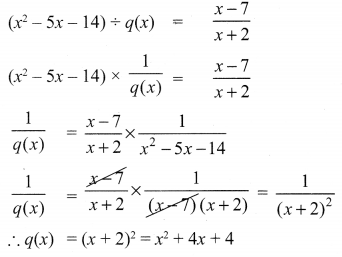You can Download Samacheer Kalvi 10th Maths Book Solutions Guide Pdf, Tamilnadu State Board help you to revise the complete Syllabus and score more marks in your examinations.

## Tamilnadu Samacheer Kalvi 10th Maths Solutions Chapter 3 Algebra Ex 3.5

10th Maths Exercise 3.5 Samacheer Kalvi Question 1.
Simplify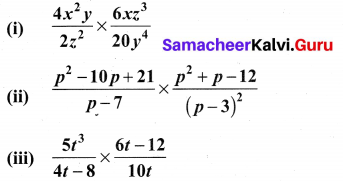Solution: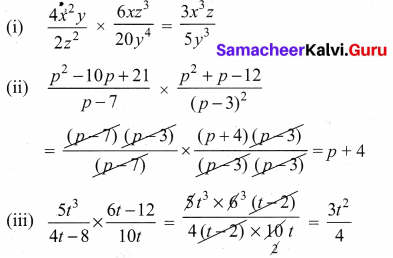Ex 3.5 Class 10 Samacheer Question 2.
Simplify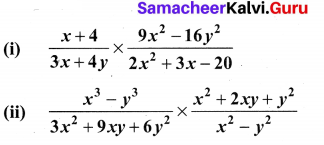Solution: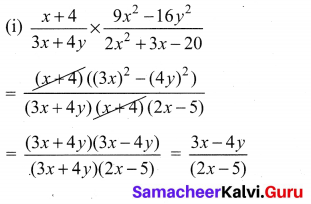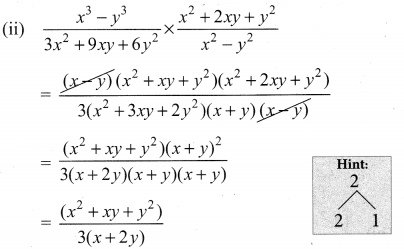10th Maths Exercise 3.5 Samacheer Question 3.
Simplify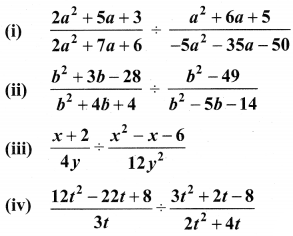Solution: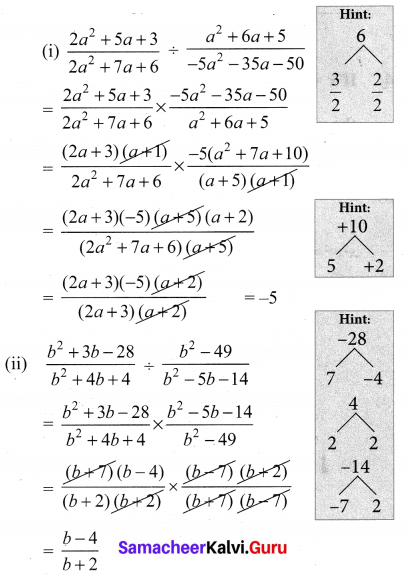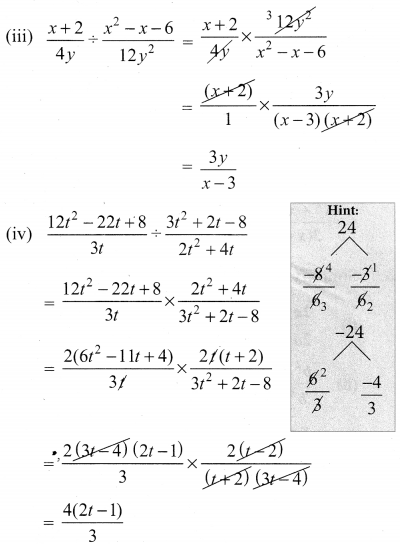10th Maths Exercise 3.5 Question 4.
If x = $$\frac{a^{2}+3 a-4}{3 a^{2}-3}$$ and y = $$\frac{a^{2}+2 a-8}{2 a^{2}-2 a-4}$$ find the value of x2y2.
Solution: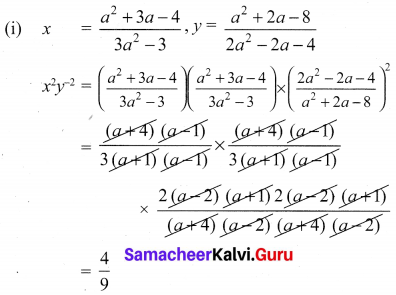Exercise 3.5 Class 10 Maths Samacheer Question 5.
If a polynomial p(x) = x2 – 5x – 14 is divided by another polynomial q(x) we get $$\frac{x-7}{x+2}$$ find q(x).
Solution:
p(x) = x2 – 5x – 14